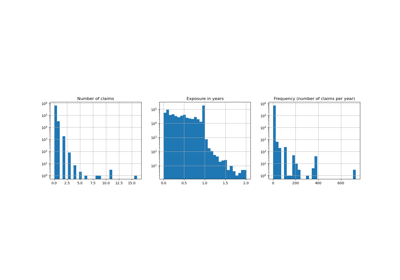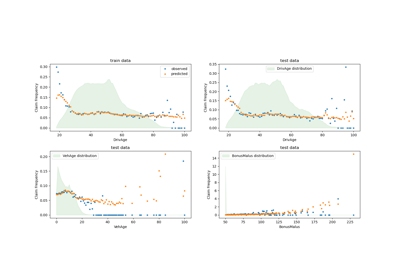# sklearn.dummy.DummyRegressor¶

class sklearn.dummy.DummyRegressor(*, strategy='mean', constant=None, quantile=None)[source]

Regressor that makes predictions using simple rules.

This regressor is useful as a simple baseline to compare with other (real) regressors. Do not use it for real problems.

Read more in the User Guide.

New in version 0.13.

Parameters:
strategy{“mean”, “median”, “quantile”, “constant”}, default=”mean”

Strategy to use to generate predictions.

• “mean”: always predicts the mean of the training set

• “median”: always predicts the median of the training set

• “quantile”: always predicts a specified quantile of the training set, provided with the quantile parameter.

• “constant”: always predicts a constant value that is provided by the user.

constantint or float or array-like of shape (n_outputs,), default=None

The explicit constant as predicted by the “constant” strategy. This parameter is useful only for the “constant” strategy.

quantilefloat in [0.0, 1.0], default=None

The quantile to predict using the “quantile” strategy. A quantile of 0.5 corresponds to the median, while 0.0 to the minimum and 1.0 to the maximum.

Attributes:
constant_ndarray of shape (1, n_outputs)

Mean or median or quantile of the training targets or constant value given by the user.

n_outputs_int

Number of outputs.

DummyClassifier

Classifier that makes predictions using simple rules.

Examples

>>> import numpy as np
>>> from sklearn.dummy import DummyRegressor
>>> X = np.array([1.0, 2.0, 3.0, 4.0])
>>> y = np.array([2.0, 3.0, 5.0, 10.0])
>>> dummy_regr = DummyRegressor(strategy="mean")
>>> dummy_regr.fit(X, y)
DummyRegressor()
>>> dummy_regr.predict(X)
array([5., 5., 5., 5.])
>>> dummy_regr.score(X, y)
0.0


Methods

 fit(X, y[, sample_weight]) Fit the random regressor. Get metadata routing of this object. get_params([deep]) Get parameters for this estimator. predict(X[, return_std]) Perform classification on test vectors X. score(X, y[, sample_weight]) Return the coefficient of determination R^2 of the prediction. set_fit_request(*[, sample_weight]) Request metadata passed to the fit method. set_params(**params) Set the parameters of this estimator. set_predict_request(*[, return_std]) Request metadata passed to the predict method. set_score_request(*[, sample_weight]) Request metadata passed to the score method.
fit(X, y, sample_weight=None)[source]

Fit the random regressor.

Parameters:
Xarray-like of shape (n_samples, n_features)

Training data.

yarray-like of shape (n_samples,) or (n_samples, n_outputs)

Target values.

sample_weightarray-like of shape (n_samples,), default=None

Sample weights.

Returns:
selfobject

Fitted estimator.

Get metadata routing of this object.

Please check User Guide on how the routing mechanism works.

Returns:

A MetadataRequest encapsulating routing information.

get_params(deep=True)[source]

Get parameters for this estimator.

Parameters:
deepbool, default=True

If True, will return the parameters for this estimator and contained subobjects that are estimators.

Returns:
paramsdict

Parameter names mapped to their values.

predict(X, return_std=False)[source]

Perform classification on test vectors X.

Parameters:
Xarray-like of shape (n_samples, n_features)

Test data.

return_stdbool, default=False

Whether to return the standard deviation of posterior prediction. All zeros in this case.

New in version 0.20.

Returns:
yarray-like of shape (n_samples,) or (n_samples, n_outputs)

Predicted target values for X.

y_stdarray-like of shape (n_samples,) or (n_samples, n_outputs)

Standard deviation of predictive distribution of query points.

score(X, y, sample_weight=None)[source]

Return the coefficient of determination R^2 of the prediction.

The coefficient R^2 is defined as (1 - u/v), where u is the residual sum of squares ((y_true - y_pred) ** 2).sum() and v is the total sum of squares ((y_true - y_true.mean()) ** 2).sum(). The best possible score is 1.0 and it can be negative (because the model can be arbitrarily worse). A constant model that always predicts the expected value of y, disregarding the input features, would get a R^2 score of 0.0.

Parameters:
XNone or array-like of shape (n_samples, n_features)

Test samples. Passing None as test samples gives the same result as passing real test samples, since DummyRegressor operates independently of the sampled observations.

yarray-like of shape (n_samples,) or (n_samples, n_outputs)

True values for X.

sample_weightarray-like of shape (n_samples,), default=None

Sample weights.

Returns:
scorefloat

R^2 of self.predict(X) w.r.t. y.

set_fit_request(*, sample_weight: Union[bool, None, str] = '$UNCHANGED$') [source]

Request metadata passed to the fit method.

Note that this method is only relevant if enable_metadata_routing=True (see sklearn.set_config). Please see User Guide on how the routing mechanism works.

The options for each parameter are:

• True: metadata is requested, and passed to fit if provided. The request is ignored if metadata is not provided.

• False: metadata is not requested and the meta-estimator will not pass it to fit.

• None: metadata is not requested, and the meta-estimator will raise an error if the user provides it.

• str: metadata should be passed to the meta-estimator with this given alias instead of the original name.

The default (sklearn.utils.metadata_routing.UNCHANGED) retains the existing request. This allows you to change the request for some parameters and not others.

New in version 1.3.

Note

This method is only relevant if this estimator is used as a sub-estimator of a meta-estimator, e.g. used inside a Pipeline. Otherwise it has no effect.

Parameters:
sample_weightstr, True, False, or None, default=sklearn.utils.metadata_routing.UNCHANGED

Metadata routing for sample_weight parameter in fit.

Returns:
selfobject

The updated object.

set_params(**params)[source]

Set the parameters of this estimator.

The method works on simple estimators as well as on nested objects (such as Pipeline). The latter have parameters of the form <component>__<parameter> so that it’s possible to update each component of a nested object.

Parameters:
**paramsdict

Estimator parameters.

Returns:
selfestimator instance

Estimator instance.

set_predict_request(*, return_std: Union[bool, None, str] = '$UNCHANGED$') [source]

Request metadata passed to the predict method.

Note that this method is only relevant if enable_metadata_routing=True (see sklearn.set_config). Please see User Guide on how the routing mechanism works.

The options for each parameter are:

• True: metadata is requested, and passed to predict if provided. The request is ignored if metadata is not provided.

• False: metadata is not requested and the meta-estimator will not pass it to predict.

• None: metadata is not requested, and the meta-estimator will raise an error if the user provides it.

• str: metadata should be passed to the meta-estimator with this given alias instead of the original name.

The default (sklearn.utils.metadata_routing.UNCHANGED) retains the existing request. This allows you to change the request for some parameters and not others.

New in version 1.3.

Note

This method is only relevant if this estimator is used as a sub-estimator of a meta-estimator, e.g. used inside a Pipeline. Otherwise it has no effect.

Parameters:
return_stdstr, True, False, or None, default=sklearn.utils.metadata_routing.UNCHANGED

Metadata routing for return_std parameter in predict.

Returns:
selfobject

The updated object.

set_score_request(*, sample_weight: Union[bool, None, str] = '$UNCHANGED$') [source]

Request metadata passed to the score method.

Note that this method is only relevant if enable_metadata_routing=True (see sklearn.set_config). Please see User Guide on how the routing mechanism works.

The options for each parameter are:

• True: metadata is requested, and passed to score if provided. The request is ignored if metadata is not provided.

• False: metadata is not requested and the meta-estimator will not pass it to score.

• None: metadata is not requested, and the meta-estimator will raise an error if the user provides it.

• str: metadata should be passed to the meta-estimator with this given alias instead of the original name.

The default (sklearn.utils.metadata_routing.UNCHANGED) retains the existing request. This allows you to change the request for some parameters and not others.

New in version 1.3.

Note

This method is only relevant if this estimator is used as a sub-estimator of a meta-estimator, e.g. used inside a Pipeline. Otherwise it has no effect.

Parameters:
sample_weightstr, True, False, or None, default=sklearn.utils.metadata_routing.UNCHANGED

Metadata routing for sample_weight parameter in score.

Returns:
selfobject

The updated object.

## Examples using sklearn.dummy.DummyRegressor¶Poisson regression and non-normal loss

Poisson regression and non-normal lossTweedie regression on insurance claims

Tweedie regression on insurance claims